Subsections

# 11.2 Counting Sort and Radix Sort

In this section we consider two sorting algorithms that are not comparison-based. These algorithms are specialized for sorting small integers. These algorithms get around the lower-bounds of Theorem 11.5 by using (parts of) the elements ofas indices into an array. Consider a statement of the form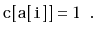This statement executes in constant time, but haspossible different outcomes, depending on the value of. This means that the execution of an algorithm that makes such a statement can not be modelled as a binary tree. Ultimately, this is the reason that the algorithms in this section are able to sort faster than comparison-based algorithms.

## 11.2.1 Counting Sort

Suppose we have an input arrayconsisting ofintegers, each in the range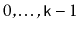. The counting-sort algorithm sortsusing an auxiliary array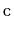of counters. It outputs a sorted version ofas an auxiliary array.

The idea behind counting-sort is simple: For each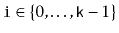, count the number of occurrences of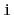inand store this in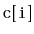. Now, after sorting, the output will look likeoccurrences of 0, followed by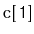occurrences of 1, followed by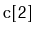occurrences of 2,..., followed byoccurrences of. The code that does this is very slick, and its execution is illustrated in Figure 11.7:

    int[] countingSort(int[] a, int k) {
int c[] = new int[k];
for (int i = 0; i < a.length; i++)
c[a[i]]++;
for (int i = 1; i < k; i++)
c[i] += c[i-1];
int b[] = new int[a.length];
for (int i = a.length-1; i >= 0; i--)
b[--c[a[i]]] = a[i];
return b;
}The firstloop in this code sets each counterso that it counts the number of occurrences ofin. By using the values ofas indices, these counters can all be computed in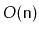time with a single for loop. At this point, we could useto fill in the output arraydirectly. However, this would not work if the elements ofhave associated data. Therefore we spend a little extra effort to copy the elements ofinto.

The nextloop, which takes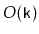time, computes a running-sum of the counters so thatbecomes the number of elements inthat are less than or equal to. In particular, for every, the output array,, will haveFinally, the algorithm scansbackwards to put its elements in order into an output array. When scanning, the element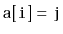is placed at locationand the value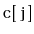is decremented.

Theorem 11..7   The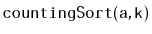method can sort an arraycontainingintegers in the set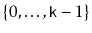intime.

The counting-sort algorithm has the nice property of being stable; it preserves the relative order of elements that are equal. If two elementsand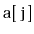have the same value, and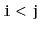thenwill appear beforein. This will be useful in the next section.

Counting-sort is very efficient for sorting an array of integers when the length,, of the array is not much smaller than the maximum value,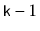, that appears in the array. The radix-sort algorithm, which we now describe, uses several passes of counting-sort to allow for a much greater range of maximum values.

Radix-sort sorts-bit integers by using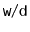passes of counting-sort to sort these integers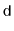bits at a time.11.2 More precisely, radix sort first sorts the integers by their least significantbits, then their next significantbits, and so on until, in the last pass, the integers are sorted by their most significantbits.

    int[] radixSort(int[] a) {
int[] b = null;
for (int p = 0; p < w/d; p++) {
int c[] = new int[1<<d];
// the next three for loops implement counting-sort
b = new int[a.length];
for (int i = 0; i < a.length; i++)
c[(a[i] >> d*p)&((1<<d)-1)]++;
for (int i = 1; i < 1<<d; i++)
c[i] += c[i-1];
for (int i = a.length-1; i >= 0; i--)
b[--c[(a[i] >> d*p)&((1<<d)-1)]] = a[i];
a = b;
}
return b;
}

(In this code, the expression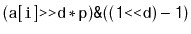extracts the integer whose binary representation is given by bitsof.) An example of the steps of this algorithm is shown in Figure 11.8.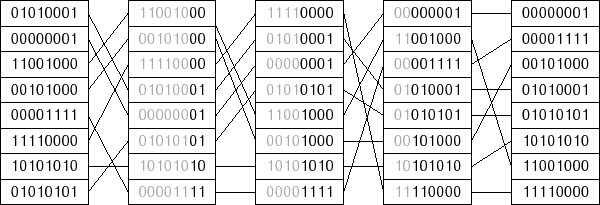This remarkable algorithm sorts correctly because counting-sort is a stable sorting algorithm. Ifare two elements ofand the most significant bit at whichdiffers fromhas index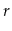, thenwill be placed beforeduring pass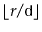and subsequent passes will not change the relative order ofand.

Radix-sort performspasses of counting-sort. Each pass requires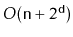time. Therefore, the performance of radix-sort is given by the following theorem.

Theorem 11..8   For any integer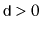, the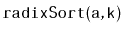method can sort an arraycontaining-bit integers in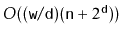time.

If we think, instead, of the elements of the array being in the range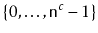, and take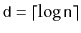we obtain the following version of Theorem 11.8.

Corollary 11..1   Themethod can sort an arraycontaininginteger values in the rangeintime.

#### Footnotes

... time.11.2
We assume thatdivides, otherwise we can always increaseto.
opendatastructures.org